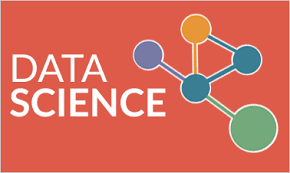11.What all things R environment includes?
1. A suite of operators for calculations on arrays, in particular matrices,
2. An effective data handling and storage facility,
3. A large, coherent, integrated collection of intermediate tools for data analysis, an effective data handling and storage facility,
4. Graphical facilities for data analysis and display either on-screen or on hardcopy, and
5. A well-developed, simple and effective programming language which includes conditionals, loops, user-defined recursive functions and input and output facilities.

12.What are the applied Machine Learning Process Steps?
1. Problem Definition: Understand and clearly describe the problem that is being solved.
2. Analyze Data: Understand the information available that will be used to develop a model.
3. Prepare Data: Define and expose the structure in the dataset.
4. Evaluate Algorithms: Develop robust test harness and baseline accuracy from which to improve and spot check algorithms.
5. Improve Results: Improve results to develop more accurate models.
6. Present Results: Details the problem and solution so that it can be understood by third parties.

13.Compare Multivariate, Univariate and Bivariate analysis?
MULTIVARIATE: Multivariate analysis focuses on the results of observations of many different variables for a number of objects.
UNIVARIATE: Univariate analysis is perhaps the simplest form of statistical analysis. Like other forms of statistics, it can be inferential or descriptive. The key fact is that only one variable is involved.
BIVARIATE: Bivariate analysis is one of the simplest forms of quantitative (statistical) analysis. It involves the analysis of two variables (often denoted as X, Y), for the purpose of determining the empirical relationship between them.

14.What is Hypothesis in Machine Learning?
The hypothesis space used  by  a  machine  learning  system  is  the  set  of  all hypotheses that might possibly be returned by it.  It is typically dened by a hypothesis language, possibly in conjunction with a language bias.

15.Differentiate between Uniform and Skewed Distribution?
UNIFORM DISTRIBUTION: A uniform distribution, sometimes also known as a rectangular distribution, is a distribution that has constant probability. The latter of which simplifies to the expected for . The continuous distribution is implemented as Uniform Distribution
SKEWED DISTRIBUTION: In probability theory and statistics, Skewness is a measure of the asymmetry of the probability distribution of a real-valued random variable about its mean. The skewness value can be positive or negative, or even undefined. The qualitative interpretation of the skew is complicated.

16.What do you understand by term Transformation in Data Acquisition?
The transformation process allows you to consolidate, cleanse, and integrate data. We can semantically arrange the data from heterogeneous sources.

17.What do you understand by term Normal Distribution?
It is a function which shows the distribution of many random variables as a symmetrical bell-shaped graph.

18.What is Data Acquisition?
It is the process of measuring an electrical or physical phenomenon such as voltage, current, temperature, pressure, or sound with a computer. A DAQ  system comprises of sensors, DAQ measurement hardware, and a computer with programmable software.

19.What is Data Collection?
Data collection is the process of collecting and measuring information on variables of interest, in a proper systematic fashion that enables one to answer stated research questions hypotheses, and revise outcomes.

20.What do you understand by term Use case?
A use case is a methodology used in system analysis to identify, clarify, and organize system requirements. The use case consists of a set of possible      sequences of interactions between systems and users in a particular environment and related to a defined particular goal.